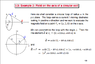# Biot-Savart Law issue

difftoint
I've been looking at my electromagnetism lecture notes I have been given and am trying to make sense of a step made in parameterising a current loop.

I am really confused about the part where dl is first state. I have no idea how the 0, sine and cosine got there. I suspect that it's something to do with arc lengths, however. Although I'm not 100% sure.

In short, I need help, desperately.

#### Attachments

•Screen shot 2010-08-30 at 02.02.51.png
17.5 KB · Views: 414

Homework Helper
Well for starters, consider a circle of radius r drawn on a two-dimensional graph, centered at the origin. Do you understand that the circle can be described as the set of points
$$(r\cos\theta, r\sin\theta)$$
for $0 \leq \theta \leq 2\pi$?

difftoint
Well for starters, consider a circle of radius r drawn on a two-dimensional graph, centered at the origin. Do you understand that the circle can be described as the set of points
$$(r\cos\theta, r\sin\theta)$$
for $0 \leq \theta \leq 2\pi$?

I understand that part with no difficulties. It explains the first step in which point rc is denoted.

It's just the second stage that I'm not seeing clearly. It looks like a(0, -sin$$\theta$$, cos$$\theta$$) is arrived at by differentiation of rc, but I cannot make the connection between doing that and finding dl.

Last edited:
qbert
there are a couple of ways to look at this.

the easiest is probably that dl is a tangent vector to the
path that the current takes. and if we have a function
r(t) = (x(t), y(t), z(t)) then the tangent vector is given
by r'(t) = (x'(t), y'(t), z'(t)).

more geometrically we can guess what the tangent vector
should look like. it's length should be the "infinitessimal" arc
so $a d\phi$ and should be pointing in the direction
the current is going. a unit vector pointing to the spot on the
circle is (0, cos, sin), so a unit vector tangent to the circle is
+/- (0, -sin, cos) where we pick the sign to match the direction
the current is going.

how did i know to pick (0, -sin, cos)? well here's an argument.
for points on the circle r^2 = a^2, so we differentiate to see
$r(\phi) \cdot \frac{dr}{d\phi} = 0$ so that r is perpendicular to
$\frac{dr}{d\phi}$. then we only need to guess something that's
perpendicular...

difftoint
there are a couple of ways to look at this.

the easiest is probably that dl is a tangent vector to the
path that the current takes. and if we have a function
r(t) = (x(t), y(t), z(t)) then the tangent vector is given
by r'(t) = (x'(t), y'(t), z'(t)).

more geometrically we can guess what the tangent vector
should look like. it's length should be the "infinitessimal" arc
so $a d\phi$ and should be pointing in the direction
the current is going. a unit vector pointing to the spot on the
circle is (0, cos, sin), so a unit vector tangent to the circle is
+/- (0, -sin, cos) where we pick the sign to match the direction
the current is going.

how did i know to pick (0, -sin, cos)? well here's an argument.
for points on the circle r^2 = a^2, so we differentiate to see
$r(\phi) \cdot \frac{dr}{d\phi} = 0$ so that r is perpendicular to
$\frac{dr}{d\phi}$. then we only need to guess something that's
perpendicular...

Thank you so much for the help. Your reply explains the solution really well.